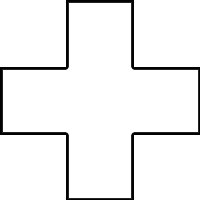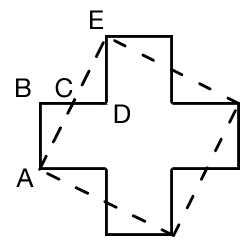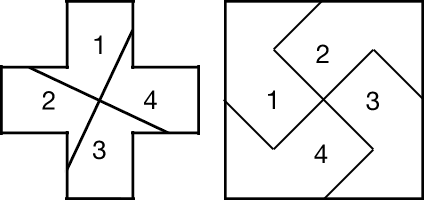1. Which is the odd man out?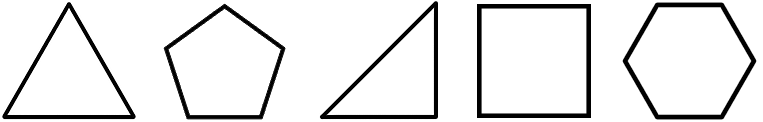Third one – non symmetrical.

2. How many triangles are there in this figure?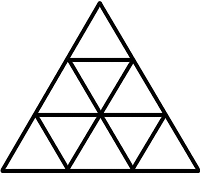13 (9 small, 3 medium, 1 big)

3. How many squares are there in the given figure? How many rectangles? (excluding squares)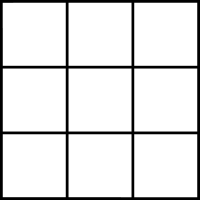14, 22

4. Can you add one more triangle to make five triangles?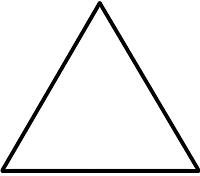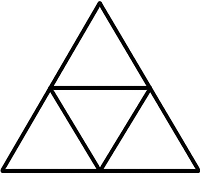5. Can you cut a hole in a piece of paper so that an elephant can pass through?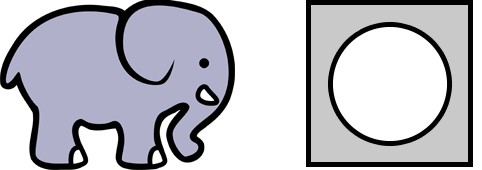Take a folded sheet of newspaper as shown and make alternate cuts as shown. Then cut along AB. This results in a long loop of paper. By making thinner cuts, the loop can be made larger.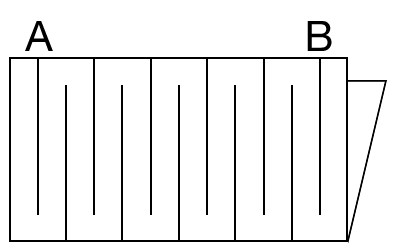6. Find the angle between the two dotted lines drawn on the surfaces of a cube.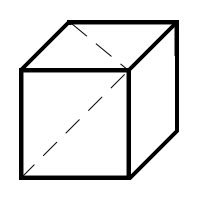60°, not 90°. Complete the triangle to see that its sides are diagonals of the faces and form an equilateral triangle.

7. (The old Hindu Puzzle) Cut the cross into 5 pieces and rearrange them so as to form a square.